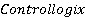### Create an Account

Home / Questions / Explain what each of the following quantities associated with a PlC timer instruction rep...

# Explain what each of the following quantities associated with a PlC timer instruction represents a Preset time b Accumulated time c TIme base 2 a When is the enable bit of a timer

Explain what each of the following quantities associated with a PlC timer instruction represents: a. Preset time

b. Accumulated time

c. TIme base

2.

a. When is the enable bit of a timer instruction true?

b. When is the timer-timing bit of a timer instruction true?

c. When does the done bit of a timer change state?

3. State the method used to reset the accumulated time of each of the following:

a. TON timer

b. TOF timer

c. RTO timer

4. Compare the way a timer is addressed in the Allen-Bradley PlC-5 and SlC·500 controllers with the method used in acontroller.

Jul 14 2020 View more View LessSubscribe To Get Solution